Next: About this document ... Up: lab_template Previous: lab_template

Subsections

# MA 1024 Lab 4: Partial derivatives, directional derivatives, and the gradient

## Purpose

The purpose of this lab is to acquaint you with using Maple to compute partial derivatives, directional derivatives, and the gradient.

## Getting Started

To assist you, there is a worksheet associated with this lab that contains examples and even solutions to some of the exercises. You can copy that worksheet to your home directory by going to your computer's Start menu and choose run. In the run field type:

\\storage\academics\math\calclab


when you hit enter, you can then choose MA1024 and then choose the worksheet

Pardiff_grad_start_C15.mw


Remember to immediately save it in your own home directory. Once you've copied and saved the worksheet, read through the background on the internet and the background of the worksheet before starting the exercises.

## Background

For a function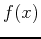of a single real variable, the derivativegives information on whether the graph ofis increasing or decreasing. Finding where the derivative is zero was important in finding extreme values. For a function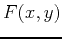of two (or more) variables, the situation is more complicated.

### Partial derivatives

A differentiable function,, of two variables has two partial derivatives:and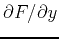. As you have learned in class, computing partial derivatives is very much like computing regular derivatives. The main difference is that when you are computing, you must treat the variable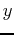as if it was a constant and vice-versa when computing.

The Maple commands for computing partial derivatives are D and diff. The Getting Started worksheet has examples of how to use these commands to compute partial derivatives.

### Classifying Stationary Points

Many applications of calculus involve finding the maximum and minimum values of functions. For example, suppose that there is a network of electrical power generating stations, each with its own cost for producing power, with the cost per unit of power at each station changing with the amount of power it generates. An important problem for the network operators is to determine how much power each station should generate to minimize the total cost of generating a given amount of power.

A crucial first step in solving such problems is being able to find and classify local extreme values of a function. What we mean by a functionhaving a local extreme value at a point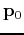is that for values ofnear,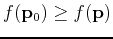for a local maximum and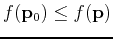for a local minimum.

In single-variable calculus, we found that we could locate candidates for local extreme values by finding points where the first derivative vanishes. For functions of two variables, the condition is that both first order partial derivatives must vanish at a local extreme value candidate point. Such a point is called a stationary point. It is also one of the three types of points called critical points. Note carefully that the condition does not say that a point where the partial derivatives vanish must be a local extreme point. Rather, it says that stationary points are candidates for local extrema. Just as was the case for functions of a single variable, there can be stationary points that are not extrema. For example, the saddle surfacehas a stationary point at the origin, but it is not a local extremum.

Finding and classifying the stationary points of a functionrequires several steps. First, the partial derivatives must be computed. Then the stationary points must be solved for by solving where both partial derivatives equal zero simultaneously.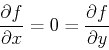Next, one must check for the presence of singular points, which might also be local extreme values. Finally, each critical point must be classified as a local maximum, local minimum, or a saddle point using the second-partials test:

Ifand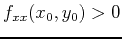thenis a local minimum.

Ifand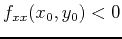thenis a local maximum.

Ifthenis a saddle point.

If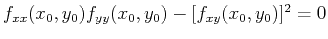then no conclusion can be made. The examples in the Getting Started worksheet are intended to help you learn how to use Maple to simplify these tasks.

### Directional Derivatives

The partial derivativesandof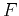can be thought of as the rate of change ofin the direction parallel to the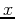andaxes, respectively. The directional derivative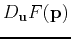, where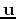is a unit vector, is the rate of change ofin the direction. There are several different ways that the directional derivative can be computed. The method most often used for hand calculation relies on the gradient, which will be described below. It is also possible to simply use the definition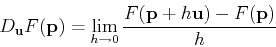to compute the directional derivative. However, the following computation, based on the definition, is often simpler to use.One way to think about this that can be helpful in understanding directional derivatives is to realize that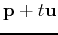is a straight line in theplane. The plane perpendicular to theplane that contains this straight line intersects the surface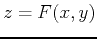in a curve whose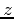coordinate is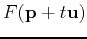. The derivative ofat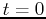is the rate of change ofat the pointmoving in the direction.

Maple doesn't have a simple command for computing directional derivatives. There is a command in the tensor package that can be used, but it is a little confusing unless you know something about tensors. Fortunately, the method described above and the method using the gradient described below are both easy to implement in Maple. Examples are given in the Getting Started worksheet.

### The Gradient

The gradient of, written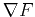, is most easily computed as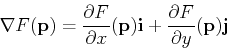As described in the text, the gradient has several important properties, including the following.
• The gradient can be used to compute the directional derivative as follows.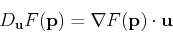• The gradient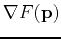points in the direction of maximum increase of the value ofat.
• The gradientis perpendicular to the level curve ofthat passes through the point.
• The gradient can be easily generalized to apply to functions of three or more variables.

Maple has a fairly simple command grad in the linalg package (which we used for curve computations). Examples of computing gradients, using the gradient to compute directional derivatives, and plotting the gradient field are all in the Getting Started worksheet.

## Exercises

For the function,
1. Using the method from the Getting Started worksheet, compute the directional derivative ofat the point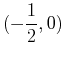in each of the directions below. Explain your results in terms of being positive, negative or zero and what that tells about the surface at that point in the given direction.
1.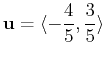2.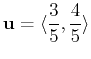3.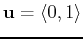2. Repeat the above exercise using only the first two directions and the points,and. What do your results suggest about the surface at these points?

3. Find and classify the stationary points forusing the second derivative test.

4. Using the method from the Getting Started worksheet, plot the gradient field and the contours ofon the same plot over the intervals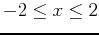and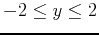. Use 30 contours, agrid and fieldstrength=fixed for the gradient plot. Describe how this plot supports your answer in the previous exercise using both the gradient field and the countour plot in your explanation.Next: About this document ... Up: lab_template Previous: lab_template
Dina J. Solitro-Rassias
2015-02-08